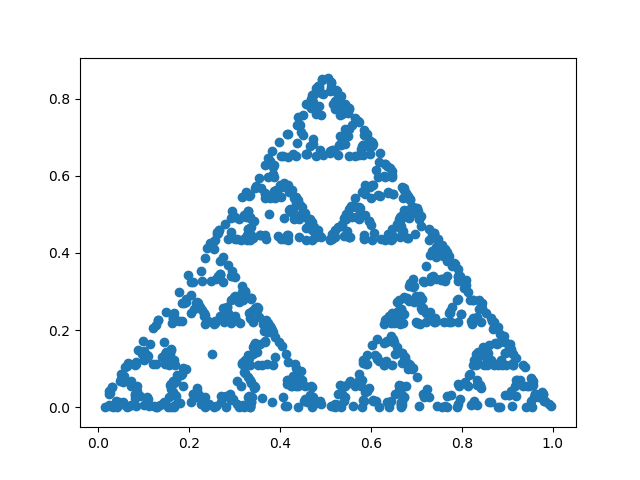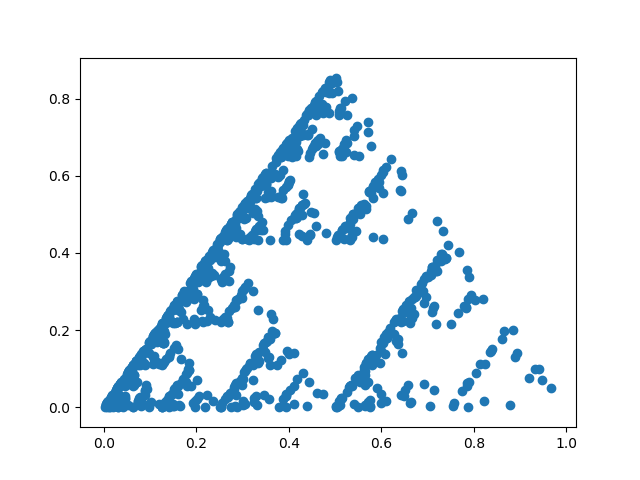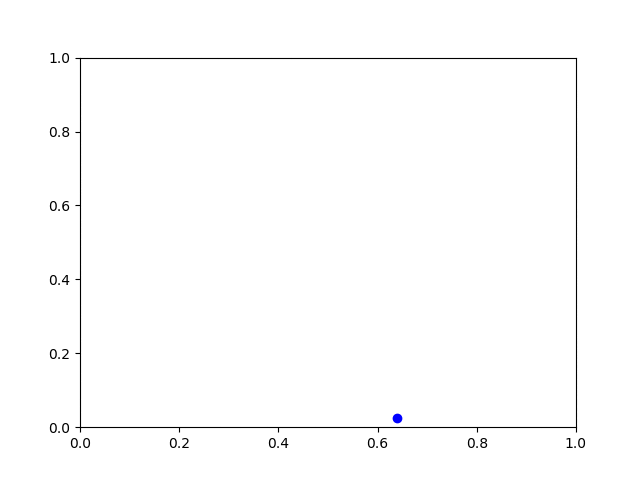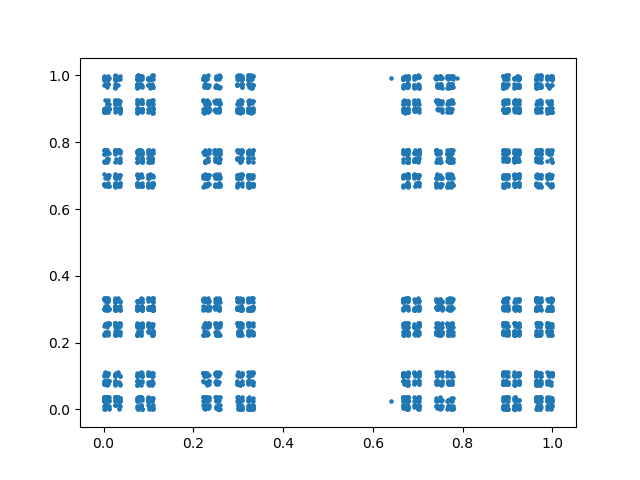# The chaos game and the Sierpinski triangle

The chaos game is played as follows. Pick a starting point at random. Then at each subsequent step, pick a triangle vertex at random and move half way from the current position to that vertex.

The result looks like a fractal called the Sierpinski triangle or Sierpinski gasket.

Here’s an example:If the random number generation is biased, the resulting triangle will show it. In the image below, the lower left corner was chosen with probability 1/2, the top with probability 1/3, and the right corner with probability 1/6.Update: Here’s an animated version that lets you watch the process in action.Here’s Python code to play the chaos game yourself.

```from scipy import sqrt, zeros
import matplotlib.pyplot as plt
from random import random, randint

def midpoint(p, q):
return (0.5*(p + q), 0.5*(p + q))

# Three corners of an equilateral triangle
corner = [(0, 0), (0.5, sqrt(3)/2), (1, 0)]

N = 1000
x = zeros(N)
y = zeros(N)

x = random()
y = random()
for i in range(1, N):
k = randint(0, 2) # random triangle vertex
x[i], y[i] = midpoint( corner[k], (x[i-1], y[i-1]) )

plt.scatter(x, y)
plt.show()
```

Update 2: Peter Norvig posted some Python code with variations on the game presented here, generalizing a triangle to other shapes. If you try the analogous procedure with a square, you simply get a square filled with random dots.

However, you can get what you might expect, the square analog of the Sierpinski triangle, the product of a Cantor set with itself, if you make a couple modifications. First, pick a side at random, not a corner. Second, move 1/3 of the way toward the chosen side, not 1/2 way.

Here’s what I got with these changes:Source: Chaos and Fractals

## 11 thoughts on “The chaos game and the Sierpinski triangle”

1. Brent Farwick

As a complete ignoramus, I get a kick out of seeing really smart guys just having fun with math.

2. Thanks!

I should have said this explicitly, but I got the game from the book referenced at the bottom of the post. I just illustrated it with the code and images.

3. Pseudonym

You can also play the chaos game with the Julia set formula, z -> z²+ c.

Inverting this formula gives you √(z – c). There are two square roots in the complex plane. Pick one at random.

4. Is there a way to quantify how fast the points converge on lying exactly on the Sierpinski triangle?

5. Christian Joseph Hansen

What if you did a star shape?

6. Francesco De Comité

A lot of variations and theory in Barnsley’s “Fractals everywhere”

7. Dave Neary

You can do a similar Chaos game for Julia sets by choosing randomly between the two square roots of a number! The inverse of the Julia iterator converges to the boundary of the julia set.
Use the mapping z -> +/- sqrt(z-c) with a random choice of the two branches of arctan on [0,2pi) and you get the wonderful result!

cx = a , cy=b, zx = 0, zy = 0; # c = a + ib, z = 0
while (1) {
tmpx = zx – cx, tmpy = zx – cy;
# Convert z into polar coordinates to make it easier to square root
r = sqrt(tmpx^2 + tmpy^2);
theta = arctan(tmpx/tmpy);
# Take square roots
new_r = sqrt(r), new_theta = theta/2;
# Choose theta/2 or theta/2 + pi as new angle randomly
if (random()%2=1) new_theta = new_theta + pi/2;
#or whatever – it’s pseudocode :-)

zx = r cos(theta); zy = r sin(theta);
plot (zx, zy);
}

8. Change the scipy import due to deprecation:

from numpy import zeros
from numpy import sqrt